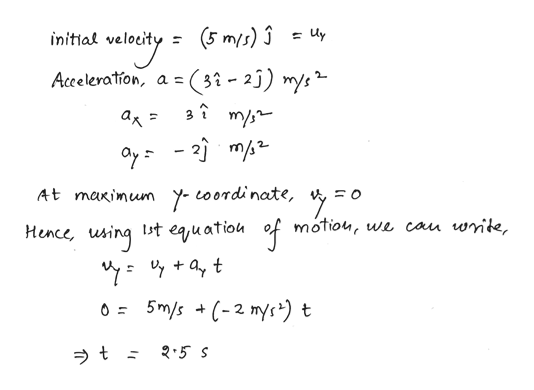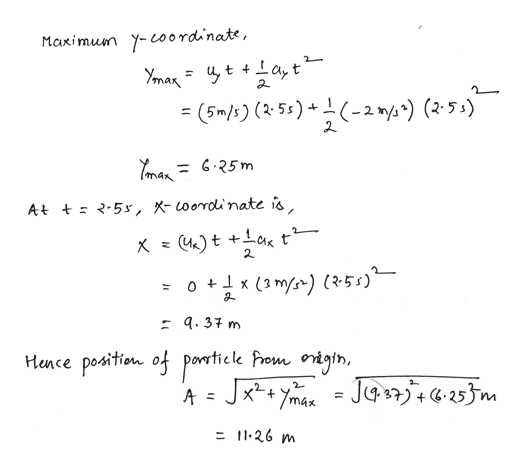# At t=0 a particle leaves the origin with a velocity of 5 m/s in the positive y direction. It's acceleration is given by a=(3i-2j)m/s^2 .at the instant the particle reaches its maximum y coordinate how far is the particle from the origin?

Question
139 views

At t=0 a particle leaves the origin with a velocity of 5 m/s in the positive y direction. It's acceleration is given by a=(3i-2j)m/s^2 .at the instant the particle reaches its maximum y coordinate how far is the particle from the origin?

check_circle

Step 1

Given:

Initial velocity along positive y direction = 5 m/s

Acceleration = (2i-3j) m/s2

Step 2

Calculating the time taken by particle to reach its maximum y-coordinate:help_outlineImage Transcriptionclose(5 m/s)3 lty = (31- 2j) mys initial Acceleration, a m/s а, oy2m2 At maximum y-coordinate, motiou, we cau wnite, Hence uning t euatiou 5m/s C-2 mys) t 0 2.5 S fullscreen
Step 3

Calculating the distance of particle from origin at the ...help_outlineImage TranscriptioncloseMaximun y-coordi'nate, (252) (5m/) (t 5s)-2 ) (25.)" Tman 6.25m 5s, X-oordli'nate is At +Lat 2 ox (3 m) (2-5s) 9.37 m Hence position of ponticlk Prom origin, JxYmaJa25}m A = = 11.26 m fullscreen

### Want to see the full answer?

See Solution

#### Want to see this answer and more?

Solutions are written by subject experts who are available 24/7. Questions are typically answered within 1 hour.*

See Solution
*Response times may vary by subject and question.
Tagged in

### Kinematics